# Stein Unbiased Risk Estimator¶

Important: Please read the installation page for details about how to install the toolboxes. $\newcommand{\dotp}{\langle #1, #2 \rangle}$ $\newcommand{\enscond}{\lbrace #1, #2 \rbrace}$ $\newcommand{\pd}{ \frac{ \partial #1}{\partial #2} }$ $\newcommand{\umin}{\underset{#1}{\min}\;}$ $\newcommand{\umax}{\underset{#1}{\max}\;}$ $\newcommand{\umin}{\underset{#1}{\min}\;}$ $\newcommand{\uargmin}{\underset{#1}{argmin}\;}$ $\newcommand{\norm}{\|#1\|}$ $\newcommand{\abs}{\left|#1\right|}$ $\newcommand{\choice}{ \left\{ \begin{array}{l} #1 \end{array} \right. }$ $\newcommand{\pa}{\left(#1\right)}$ $\newcommand{\diag}{{diag}\left( #1 \right)}$ $\newcommand{\qandq}{\quad\text{and}\quad}$ $\newcommand{\qwhereq}{\quad\text{where}\quad}$ $\newcommand{\qifq}{ \quad \text{if} \quad }$ $\newcommand{\qarrq}{ \quad \Longrightarrow \quad }$ $\newcommand{\ZZ}{\mathbb{Z}}$ $\newcommand{\CC}{\mathbb{C}}$ $\newcommand{\RR}{\mathbb{R}}$ $\newcommand{\EE}{\mathbb{E}}$ $\newcommand{\Zz}{\mathcal{Z}}$ $\newcommand{\Ww}{\mathcal{W}}$ $\newcommand{\Vv}{\mathcal{V}}$ $\newcommand{\Nn}{\mathcal{N}}$ $\newcommand{\NN}{\mathcal{N}}$ $\newcommand{\Hh}{\mathcal{H}}$ $\newcommand{\Bb}{\mathcal{B}}$ $\newcommand{\Ee}{\mathcal{E}}$ $\newcommand{\Cc}{\mathcal{C}}$ $\newcommand{\Gg}{\mathcal{G}}$ $\newcommand{\Ss}{\mathcal{S}}$ $\newcommand{\Pp}{\mathcal{P}}$ $\newcommand{\Ff}{\mathcal{F}}$ $\newcommand{\Xx}{\mathcal{X}}$ $\newcommand{\Mm}{\mathcal{M}}$ $\newcommand{\Ii}{\mathcal{I}}$ $\newcommand{\Dd}{\mathcal{D}}$ $\newcommand{\Ll}{\mathcal{L}}$ $\newcommand{\Tt}{\mathcal{T}}$ $\newcommand{\si}{\sigma}$ $\newcommand{\al}{\alpha}$ $\newcommand{\la}{\lambda}$ $\newcommand{\ga}{\gamma}$ $\newcommand{\Ga}{\Gamma}$ $\newcommand{\La}{\Lambda}$ $\newcommand{\si}{\sigma}$ $\newcommand{\Si}{\Sigma}$ $\newcommand{\be}{\beta}$ $\newcommand{\de}{\delta}$ $\newcommand{\De}{\Delta}$ $\newcommand{\phi}{\varphi}$ $\newcommand{\th}{\theta}$ $\newcommand{\om}{\omega}$ $\newcommand{\Om}{\Omega}$

This tour uses the Stein Unbiased Risk Estimator (SURE) to optimize the value of parameters in denoising algorithms.

In :
warning('off')
warning('on')


## Denoising and SURE¶

We consider a simple generative model of noisy images $F = f_0+W$ where $f_0 \in \RR^N$ is a deterministic image of $N$ pixels, and $W$ is a Gaussian white noise distributed according to $\Nn(0,\si^2 \text{Id}_N)$, where $\si^2$ is the variance of noise.

The goal of denoising is to define an estimator $h(F)$ of $f_0$ that depends only on $F$, where $h : \RR^N \rightarrow \RR^N$ is a potentially non-linear mapping.

Note that while $f_0$ is a deterministic image, both $F$ and $h(F)$ are random variables (hence the capital letters).

The goal of denoising is to reduce as much as possible the denoising error given some prior knowledge on the (unknown) image $f_0$. A mathematical way to measure this error is to bound the quadratic risk $\EE_W(\norm{h(F) - f_0}^2)$, where the expectation is computed with respect to the distribution of the noise $W$

For real life applications, one does not have access to the underlying image $f_0$. In this tour, we however assume that $f_0$ is known, and $f = f_0 + w\in \RR^N$ is generated using a single realization of the noise $w$ that is drawn from $W$. We define the estimated deterministic image as $h(f)$ which is a realization of the random vector $h(F)$.

Number $N = n \times n$ of pixels.

In :
n = 128*2;
N = n^2;


First we load an image $f \in \RR^N$ where $N=n \times n$ is the number of pixels.

In :
name = 'hibiscus';


Display it.

In :
clf;
imageplot(f0);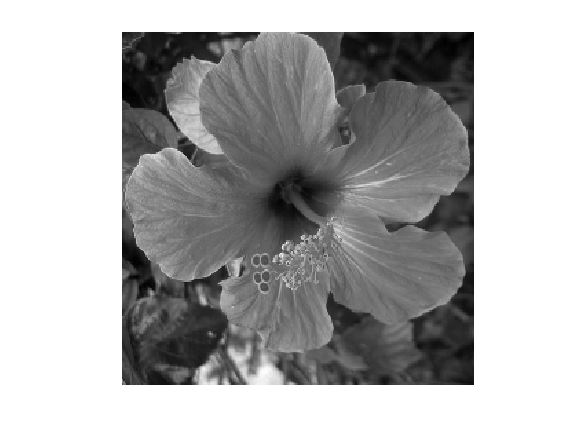Standard deviation $\si$ of the noise.

In :
sigma = .08;


Then we add Gaussian noise $w$ to obtain $f=f_0+w$.

In :
f = f0 + sigma*randn(n);


Display the noisy image. Note the use of the |clamp| function to saturate the result to $[0,1]$ to avoid a loss of contrast of the display.

In :
clf;
imageplot(clamp(f), strcat(['Noisy, SNR=' num2str(snr(f0,f),3) 'dB']));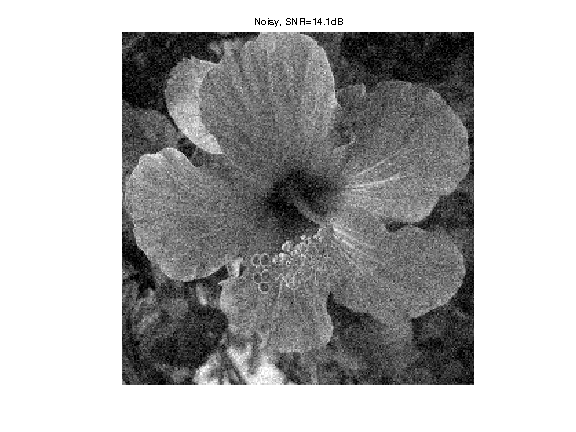The Stein Unbiased Risk Estimator (SURE) associated to the mapping $h$ is defined as $$\text{SURE}(f) = -N\si^2 + \norm{h(f)-f}^2 + 2\si^2 \text{df}(f)$$ where df stands for degree of freedom, and is defined as $$\text{df}(f) = \text{div} h(f) = \sum_i \pd{h}{f_i}(f).$$

It has been introduced in:

Stein, Charles M. (November 1981). "Estimation of the Mean of a Multivariate Normal Distribution". The Annals of Statistics 9 (6): 1135-1151.

And it has been applied to wavelet-based non-linear denoising in:

Donoho, David L.; Iain M. Johnstone (December 1995). "Adapting to Unknown Smoothness via Wavelet Shrinkage". Journal of the American Statistical Association (Journal of the American Statistical Association, Vol. 90, No. 432) 90 (432): 1200-1244.

If the mapping $f \mapsto h(f)$ is differentiable outside a set of zero measure (or more generally weakly differentiable), then SURE defines an unbiased estimate of the quadratic risk $$\EE_W(\text{SURE}(F)) = \EE_W( \norm{f_0-h(F)}^2 ).$$ This is especially useful, since the evaluation of SURE does not necessitate the knowledge of the clean signal $f_0$ (but note however that it requires the knowledge of the noise level $\si$).

In practice, one replaces $\text{SURE}(F)$ from its empirical evaluation $\text{SURE}(f)$ on a single realization $f$. One can then minimize $\text{SURE}(f)$ with respect to a parameter $\la$ that parameterizes the denoiser $h=h_\la$.

## Linear Denoising SURE¶

We consider a translation-invariant linear denoising operator, which is thus a convolution $$h(f) = f \star g$$ where $g \in \RR^N$ is a low pass kernel, and $\star$ denotes the periodic 2-D convolution.

Since we use periodic boundary condition, we compute the convolution as a multiplication over the Fourier domain. $$\forall \om, \quad \hat h(f)(\om) = \hat f(\om) \hat g(\om)$$ where $\hat g(\om)$ is the frequency $\om$ of the discrete 2-D Fourier transform of $g$ (computed using the function |fft2|).

In :
convol = @(f,g)real(ifft2(fft2(f) .* repmat(fft2(g), [1 1 size(f,3)]) ));


We define a parameteric kernel $g_\la$ parameterized by its bandwidth $\la>0$. We use here a Gaussian kernel $$g_\la(a) = \frac{1}{Z_\la} e^{ -\frac{\norm{a}}{2 \la^2} }$$ where $Z_\la$ ensures that $\sum_a g_\la(a) = 1$.

In :
normalize = @(f)f/sum(f(:));
x = [0:n/2 -n/2+1:-1];
[Y,X] = meshgrid(x,x);
g = @(lambda)normalize( exp( -(X.^2+Y.^2)/(2*lambda^2) ) );


Define our denoising operator $h=h_\la$ (we make explicit the dependency on $\la$): $$h_\la(f) = g_\la \star f.$$

In :
h = @(f,lambda)convol(f, g(lambda));


Example of denoising result.

In :
lambda = 1.5;
clf;
imageplot(clamp(h(f,lambda)));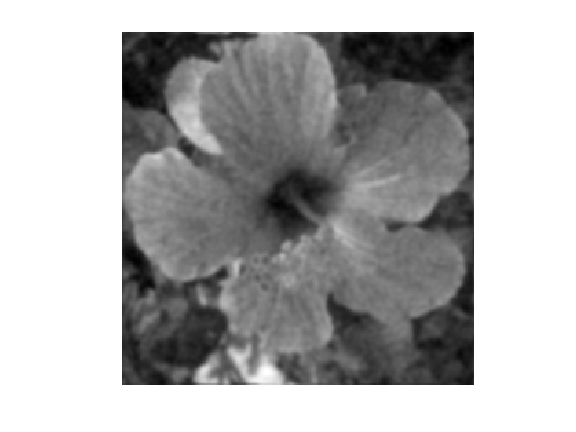For linear operator, the dregree of freedom is equal to the trace of the operator, and thus in our case it is equal to the sum of the Fourier transform $$\text{df}_\la(f) = \text{tr}(h_\la) = \sum_{\om} \hat g_\la(\om)$$ Note that we have made explicit the dependency of df with respect $\la$. Note also that df$(f)$ actually does not depends on $f$.

In :
df = @(lambda)real(sum(sum(fft2(g(lambda)))));


We can now define the SURE=SURE$_\la$ operator, as a function of $f, h(f), \lambda$.

In :
SURE = @(f,hf,lambda)-N*sigma^2 + norm(hf-f, 'fro')^2 + 2 * sigma^2 * df(lambda);


Exercise 1

For a given $\lambda$, display the histogram of the repartition of the quadratic error $\norm{y-h(y)}^2$ and of $\text{SURE}(y)$. Compute these repartition using Monte-Carlo simulation (you need to generate lots of different realization of the noise $W$. Display in particular the location of the mean of these quantities. ean isplay

In :
exo1()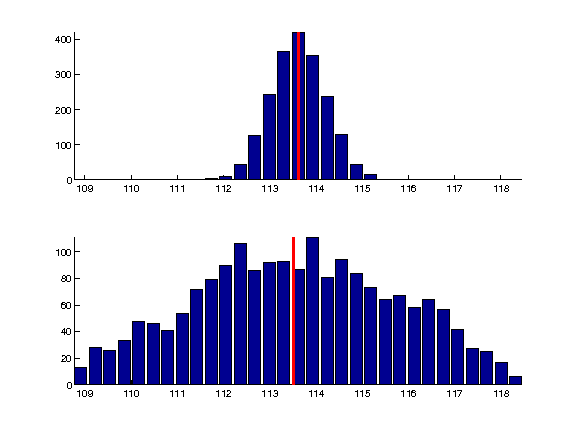In :
%% Insert your code here.


In practice, the SURE is used to set up the value of $\la$ from a single realization $f=f_0+w$, by minimizing $\text{SURE}_\la(f)$.

Exercise 2

Compute, for a single realization $f=f_0+w$, the evolution of $$E(\la) = \text{SURE}_\la(f) \qandq E_0(\lambda) = \norm{f-h_\la(f)}^2$$ as a function of $\lambda$.

In :
exo2()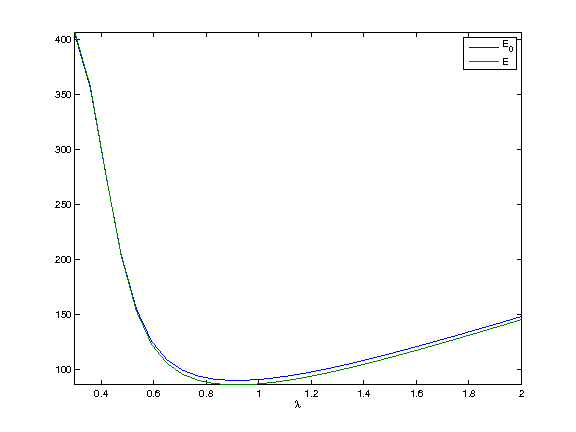In :
%% Insert your code here.


Exercise 3

Display the best denoising result $h_{\la^*}(f)$ where $$\la^* = \uargmin{\la} \text{SURE}_\la(f)$$

In :
exo3()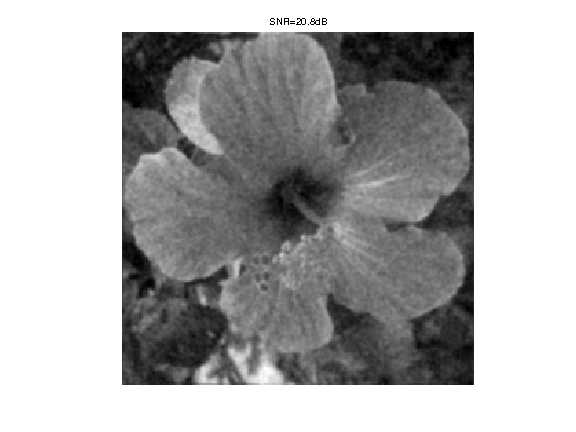In :
%% Insert your code here.


## Soft Thresholding SURE¶

In order to enhance the denoising results for piecewise regular signal and image, it is possible to use non-linear thresholding in an orthogonal wavelet basis $\Bb = \{ \psi_m \}_{m}$ where $\psi_m \in \RR^N$ is a wavelet element.

Re-generate a noisy image.

In :
f = f0 + sigma*randn(n);


The soft-thresholding estimator thus reads $$h_\la(f) = \sum_m s_\la( \dotp{f}{\psi_m} ) \psi_m \qwhereq s_\la(\al) = \max\pa{0, 1-\frac{\la}{\abs{\al}}} \al.$$ It can be conveniently written as $$h_\la = \Ww^* \circ S_\la \circ \Ww$$ where $\Ww$ and $\Ww^*$ are forward and inverse wavelet transform $$\Ww(f) = ( \dotp{f}{\psi_m} )_m \qandq \Ww^*(x) = \sum_m x_m \psi_m,$$ and $S_\la$ is the diagonal soft thresholding operator $$S_\la(x) = ( s_\la(x_m) )_m.$$

Define the wavelet transform and its inverse.

In :
W  = @(f)perform_wavortho_transf(f,0,+1);
Ws = @(x)perform_wavortho_transf(x,0,-1);


Display the wavelet transform $\Ww(f_0)$ of the original image.

In :
clf;
plot_wavelet(W(f0),1);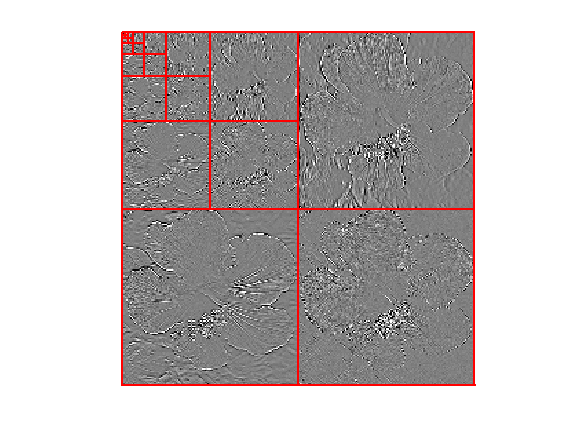Define the soft thresholding operator.

In :
S = @(x,lambda)max(0, 1-lambda ./ max(1e-9,abs(x)) ) .* x;


Define the denoising operator.

In :
h = @(f,lambda)Ws(S(W(f),lambda));


Example of denoising result.

In :
lambda = 3*sigma/2;
clf;
imageplot(clamp(h(f,lambda)));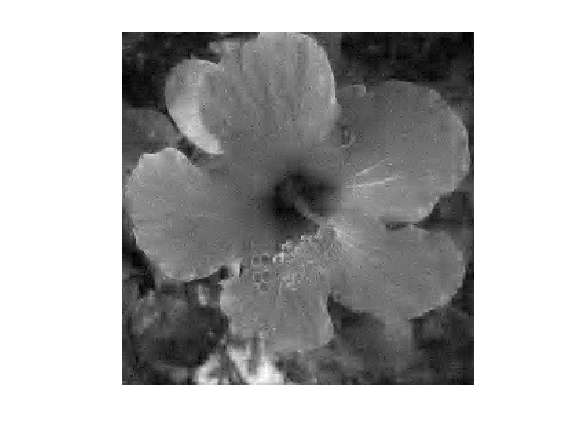Since $Ww$ is an orthogonal transform, one has $$\text{df}(f) = \text{div}( S_\la )( \Ww(f) ) = \sum_m s_\la'( \dotp{f}{\psi_m} ) = \norm{\Ww(h(f))}_0$$ where $s_\la'$ is the derivative of the 1-D function $s_\la$, and $\norm{\cdot}_0$ is the $\ell^0$ pseudo-norm $$\norm{x}_0 = \abs{ \enscond{m}{x_m \neq 0} }.$$

To summerize, the degree of freedom is equal to the number of non-zero coefficients in the wavelet coefficients of $h(f)$.

In :
df = @(hf,lambda)sum(sum( abs(W(hf))>1e-8 ));


We can now define the SURE operator, as a function of $f, h(f), \lambda$.

In :
SURE = @(f,hf,lambda)-N*sigma^2 + norm(hf-f, 'fro')^2 + 2 * sigma^2 * df(hf,lambda);


Exercise 4

For a given $\lambda$, display the histogram of the repartition of the quadratic error $\norm{y-h(y)}^2$ and of $\text{SURE}(y)$. Compute these repartition using Monte-Carlo simulation (you need to generate lots of different realization of the noise $W$. Display in particular the location of the mean of these quantities. Hint: you can do the computation directly over the wavelet domain, i.e. consider that the noise is added to the wavelet transform. ean isplay

In :
exo4()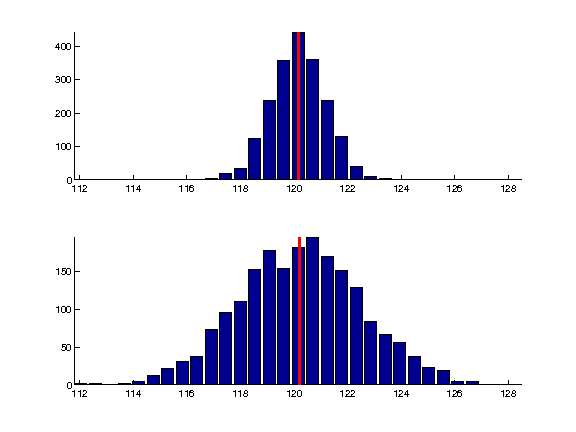In :
%% Insert your code here.


Exercise 5

Compute, for a single realization $f=f_0+w$, the evolution of $$E(\la) = \text{SURE}_\la(f) \qandq E_0(\lambda) = \norm{f-h_\la(f)}^2$$ as a function of $\lambda$.

In :
exo5()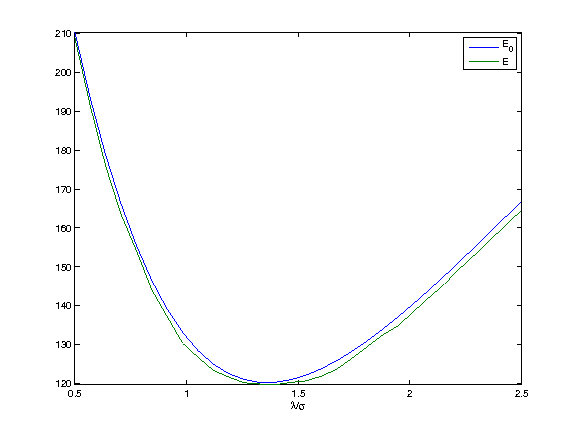In :
%% Insert your code here.


Exercise 6

Display the best denoising result $h_{\la^*}(f)$ where $$\la^* = \uargmin{\la} \text{SURE}_\la(f)$$

In :
exo6()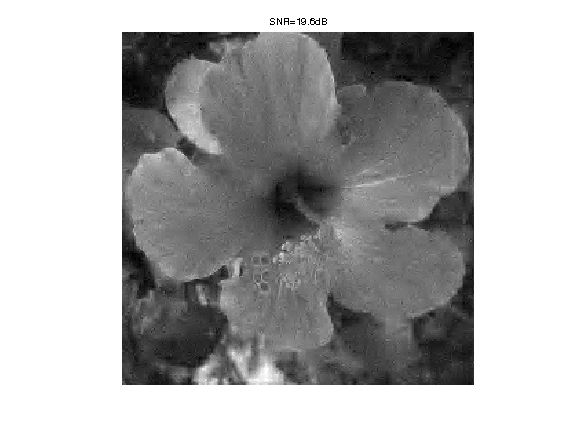In :
%% Insert your code here.


## Block-soft Thresholding SURE¶

To improve the result of soft thresholding, it is possible to threshold blocks of coefficients.

We define a partition $\{1,\ldots,N\} = \cup_k b_k$ of the set of wavelet coefficient indexes. The block thresholding is defined as $$h_\la(f) = \sum_k \sum_{m \in b_k} a_\la( e_k ) \dotp{f}{\psi_m} \psi_m \qwhereq e_k = \sum_{m \in b_k} \abs{\dotp{f}{\psi_m}}^2,$$ where we use the James-Stein attenuation threshold $$a_\la(e) = \max\pa{ 0, 1 - \frac{\la^2}{e^2} }.$$

The block size $q$.

In :
q = 4;


A function to extract blocks.

In :
[dX,dY,X,Y] = ndgrid(0:q-1,0:q-1,1:q:n-q+1,1:q:n-q+1);
I = X+dX + (Y+dY-1)*n;
blocks = @(fw)reshape(fw(I(:)),size(I));


A function to reconstruct an image from blocks.

In :
linearize = @(x)x(:);
unblock = @(H)reshape( accumarray( I(:), linearize(H), [n*n 1], @min), [n n]);


Compute the average energy of each block, and duplicate.

In :
energy = @(H)mean(mean(abs(H).^2,1),2);
energy = @(H)repmat( max3(energy(H),1e-15), [q q]);


Threshold the blocks. We use here a Stein block thresholding. All values within a block are atenuated by the same factor.

In :
S = @(H,lambda)max(1-lambda^2 ./ energy(H),0) .* H;


Block thresholding estimator $h_\lambda(f)$.

In :
h = @(f,lambda)Ws(unblock(S(blocks(W(f)),lambda) ) );


Example of block denoising.

In :
lambda = 1.1*sigma;
clf;
imageplot(clamp(h(f,lambda)));